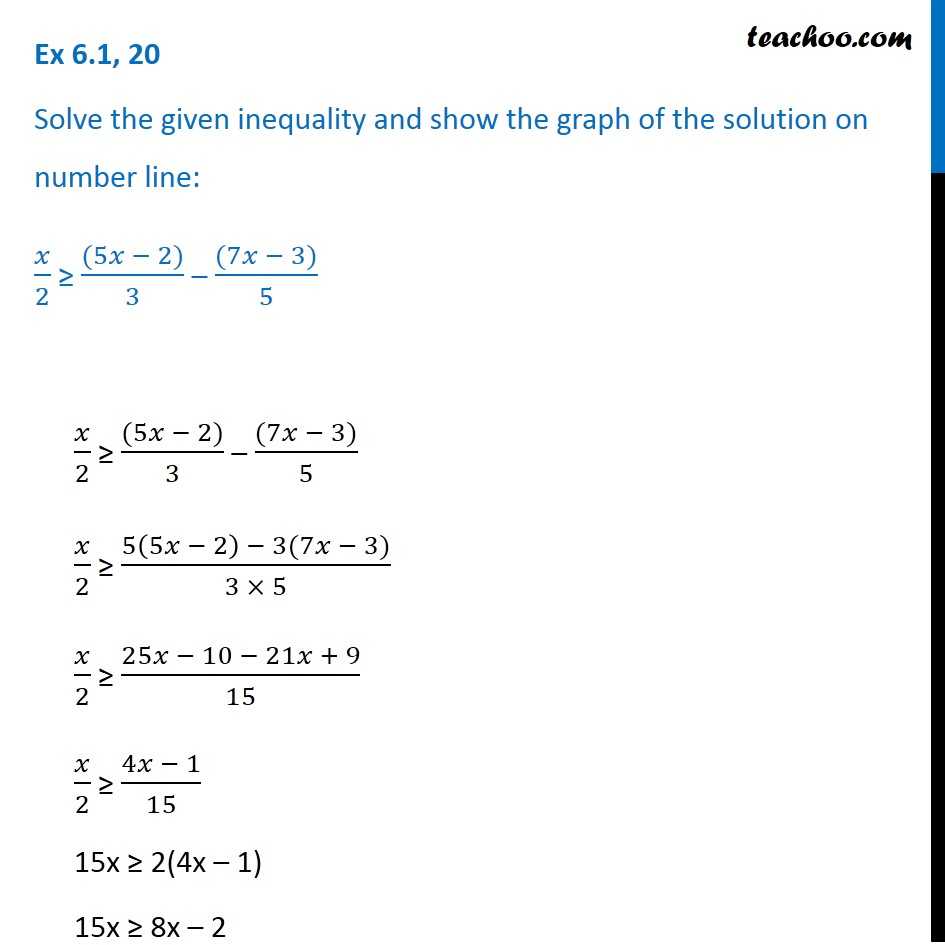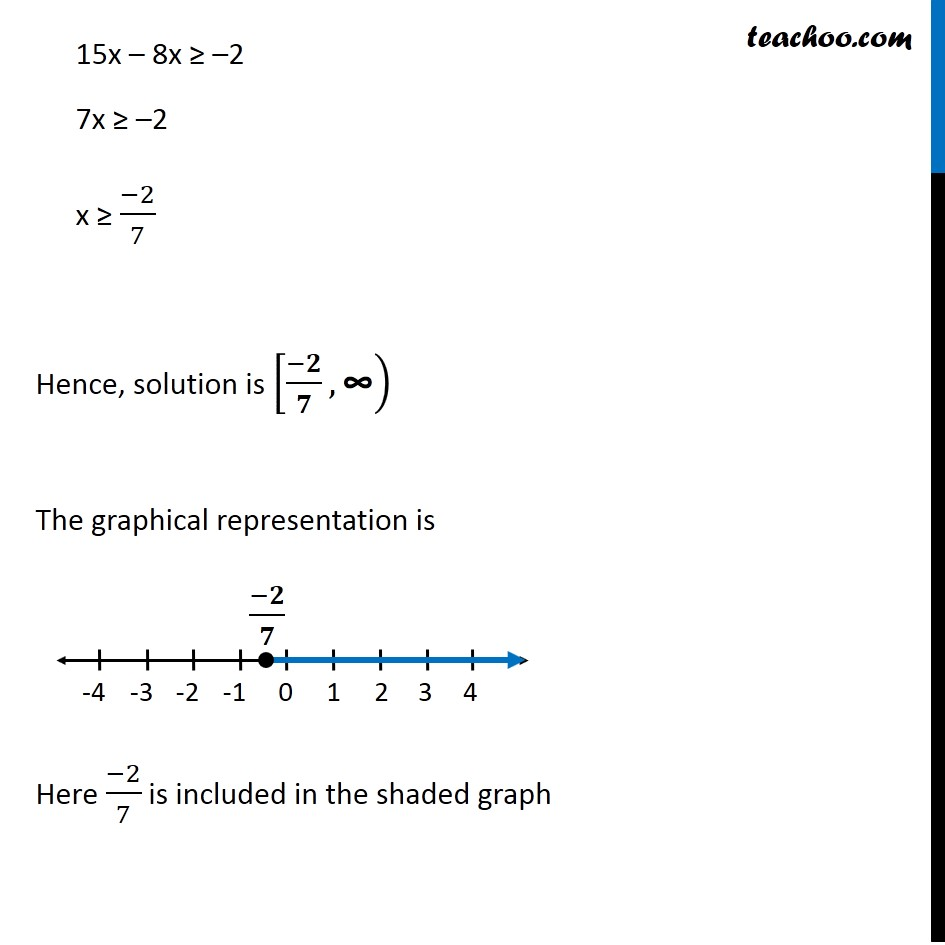Ex 6.1

Chapter 6 Class 11 Linear Inequalities (Term 2)
Serial order wise### Transcript

Ex 6.1, 20 Solve the given inequality and show the graph of the solution on number line: 𝑥/2 ≥ ((5𝑥 − 2))/3 – ((7𝑥 − 3))/5 𝑥/2 ≥ ((5𝑥 − 2))/3 – ((7𝑥 − 3))/5 𝑥/2 ≥ (5(5𝑥 − 2) − 3(7𝑥 − 3))/(3 × 5) 𝑥/2 ≥ (25𝑥 − 10 − 21𝑥 + 9)/15 𝑥/2 ≥ (4𝑥 − 1)/15 15x ≥ 2(4x – 1) 15x ≥ 8x – 2 15x – 8x ≥ –2 7x ≥ –2 x ≥ (−2)/7 Hence, solution is [(−𝟐)/𝟕,"∞" ) The graphical representation is Here (−2)/7 is included in the shaded graph